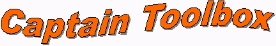The Captain Toolbox is a set of MATLAB ® functions for non-stationary time series analysis and forecasting. Following successful beta-testing, an evaluation version is now available for download from this web page.

### Matlab

MATLAB ® is a high performance numeric computation and visualization software package available from The Mathworks Inc. The flexible Matlab environment allows for straightforward integration with specialist toolboxes such as Captain.

### Toolbox history

The estimation algorithms were developed originally in the 1960/1970/1980's for the CAPTAIN and microCAPTAIN time series analysis and forecasting packages (MS-DOS based). The associated optimisation algorithms were developed in the 1980/1990's and are used in the latest version of microCAPTAIN. The MATLAB ® implementation is much more flexible than microCAPTAIN and includes the latest innovations and improvements to the algorithms.

### Toolbox applications

At present, the toolbox consists of two main subsets. In the first place, the Time Variable Parameter (TVP) subset allows for the optimal estimation of dynamic regression models, including harmonic regression, linear regression and autoregression. Secondly, the Input-Output subset provides for robust unbiased estimation of multivariable discrete and continuous time transfer function models. The toolbox is useful for signal extraction, interpolation, backcasting, forecasting and data-based mechanistic (DBM) analysis of a wide range of linear and non-linear stochastic systems. One advantage of the DBM model is its simplicity and ability to characterise the dominant modal behaviour of a dynamic system. This makes such a model an ideal basis for model-based control system design.

### Toolbox authorsc.taylor@lancaster.ac.uk 23-FEB-2011